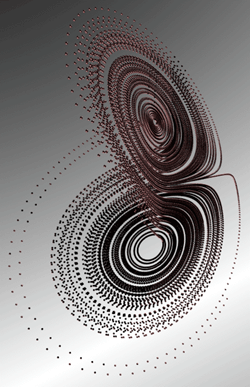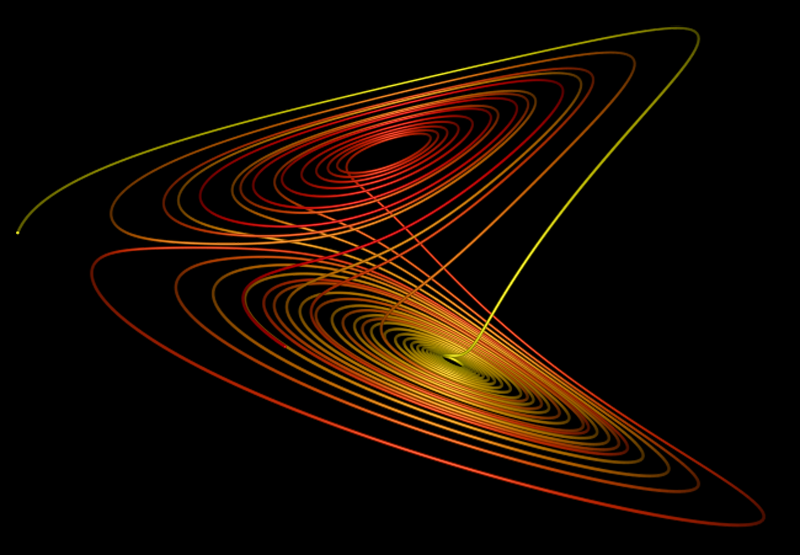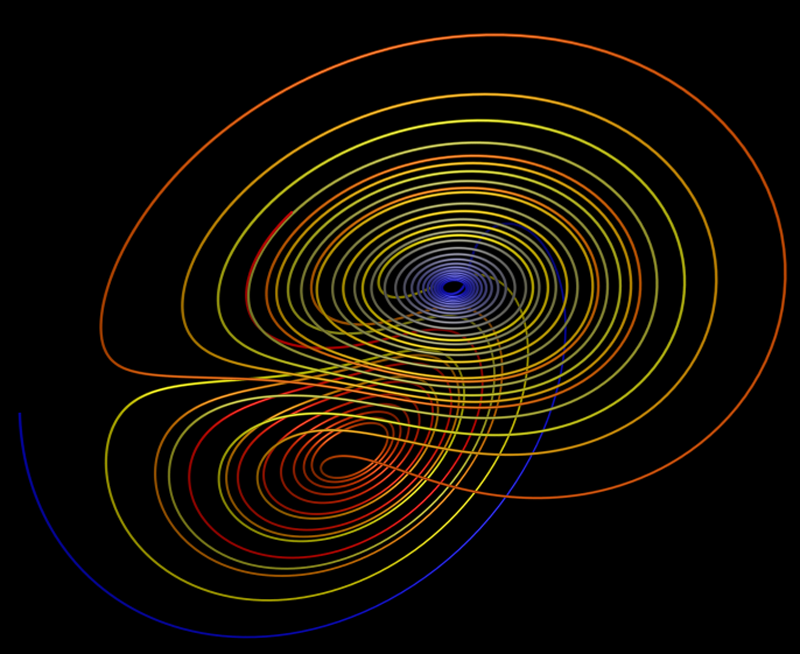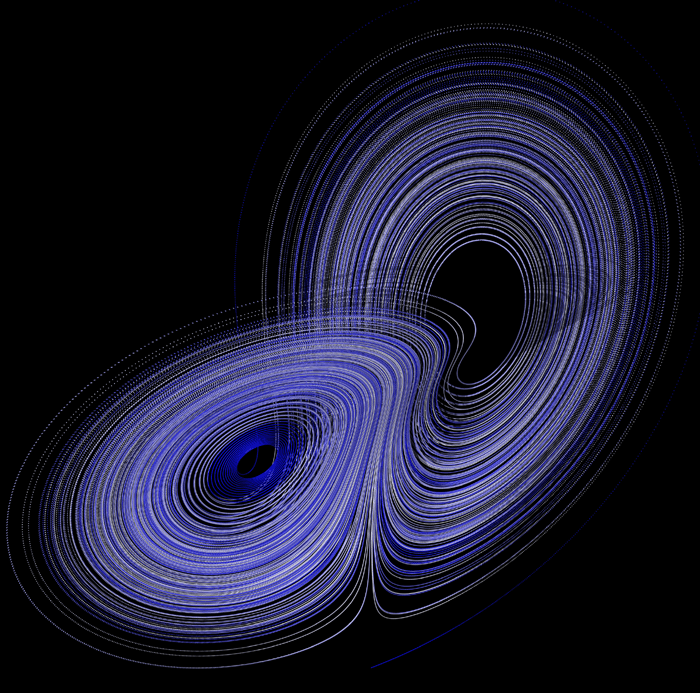# The Lorenz Attractor in 3D

Images by Paul Bourke
April 1997

MSWindows application by Dominic van Berkel.

Listen (mov or midi) to the Lorenz attractor.
The three axes are each mapped to a different instrument.

lorenz.m4v for an iPod-video.

 This image appeared in the Nature journal 31 August 2000, pp 949 as part of an article titled The Lorenz Attractor Exists, written by Ian Stewart. It was created as part of an OpenGL interactive viewer and rendered on a farm of Dec Alphas using PovRay. Appeared in the book "What Shape is a Snowflake" by Ian Stewart, page 177. Appeared in Wiedzaizycie #7, July 2003, page 49. Appeared on a poster for the Second DANCE's Winter School: Recent Trends in Nonlinear Science Castellon, January 2005The lorenz attractor was first studied by Ed N. Lorenz, a meteorologist, around 1963. It was derived from a simplified model of convection in the earth's atmosphere. It also arises naturally in models of lasers and dynamos. The system is most commonly expressed as 3 coupled non-linear differential equations.

dx / dt = a (y - x)

dy / dt = x (b - z) - y

dz / dt = xy - c z

One commonly used set of constants is a = 10, b = 28, c = 8 / 3. Another is a = 28, b = 46.92, c = 4. "a" is sometimes known as the Prandtl number and "b" the Rayleigh number.

The series does not form limit cycles nor does it ever reach a steady state. Instead it is an example of deterministic chaos. As with other chaotic systems the Lorenz system is sensitive to the initial conditions, two initial states no matter how close will diverge, usually sooner rather than later.

Typical traces of each coordinate are shown belowWhile the equations look simple enough they lead to wondrous trajectories, some examples of which are illustrated below.A physical model simulating the Lorenz equations has been attributed to Willem Malkus and Lou Howard around 1970. It consists of leaking cups on the rim of a larger wheel as shown in the diagram on the right. Liquid flows from the pipe at the top, each cup leaks from the bottom. Under different input flow rates you should be able to convince yourself that under just the right flow rate the wheel will spin one way and then the other chaotically. Of course you can always build it.....C source ```#include "stdio.h" #include "stdlib.h" #include "math.h" #define N 10000 int main(int argc,char **argv) { int i=0; double x0,y0,z0,x1,y1,z1; double h = 0.01; double a = 10.0; double b = 28.0; double c = 8.0 / 3.0; x0 = 0.1; y0 = 0; z0 = 0; for (i=0;i 100) printf("%d %g %g %g\n",i,x0,y0,z0); } } ```PovRay 3.5 macro by Marcus Fritzsch

```// N = number iterations
// h, a, b, c: initial parameters
// x0, y0, z0: start-location

#macro lorenz(h, a, b, c, x0, y0, z0, N, rad)
// use it like:
// lorenz(0.001099, 10, 28, 8/3, 0.0001, 0.0001, 0.0001, 350000, 0.04)
#local i = 0;
union {
#while (i < N)
#local x1 = x0 + h * a * (y0 - x0);
#local y1 = y0 + h * (x0 * (b - z0) - y0);
#local z1 = z0 + h * (x0 * y0 - c * z0);
#if (i > 100)
sphere {
pigment { color rgb <i/N,i/N,1> }
}
#end
#local i = i + 1;
#local x0 = x1;
#local y0 = y1;
#local z0 = z1;
#end
}
#end
```

PovRay rendering by Marcus FritzschThe waterwheel actually built by Planeten Paultje for the Dutch Annual Physics Teacher Conference in December 2005, the "Woudschotenconferentie Natuurkunde 2005".Experimental setupSnapshot of chart recorder

Second Life example

```default {
state_entry() {
llOwnerSay("Touch to start build");
}

touch_start(integer total_number) {
integer i;
integer N = 3000;
integer counter = 0;
float a = 10;
float b = 28;
float c = 8/3.0;
float h = 0.01;
vector p0 = <0.1,0,0>;
vector plast = p0;
vector p1;
vector offset = <0,0,-1>;
float scalefactor = 0.15;

offset += llGetPos();

for (i=0;counter<N;i++) {

p1.x = p0.x + h * a * (p0.y - p0.x);
p1.y = p0.y + h * (p0.x * (b - p0.z) - p0.y);
p1.z = p0.z + h * (p0.x * p0.y - c * p0.z);
llOwnerSay((string)i + " <" + (string)p1.x + "," + (string)p1.y + "," + (string)p1.z +">");

if (i > 100) {
//llRezObject("ball", p1+offset, ZERO_VECTOR, ZERO_ROTATION, 1);
rotation rot = llRotBetween(<0,0,1>,scalefactor*(p1-plast));
integer len = (integer)(100*llVecMag(scalefactor*(p1-plast)));
if (len > 10) {
llRezObject("lineseg", offset + scalefactor*(p1+plast)/2, ZERO_VECTOR, rot, len);
plast = p1;
counter++;
}
} else {
plast = p1;
}
p0 = p1;
}
}
}
```Sculpture in Vevey, Switzerland